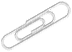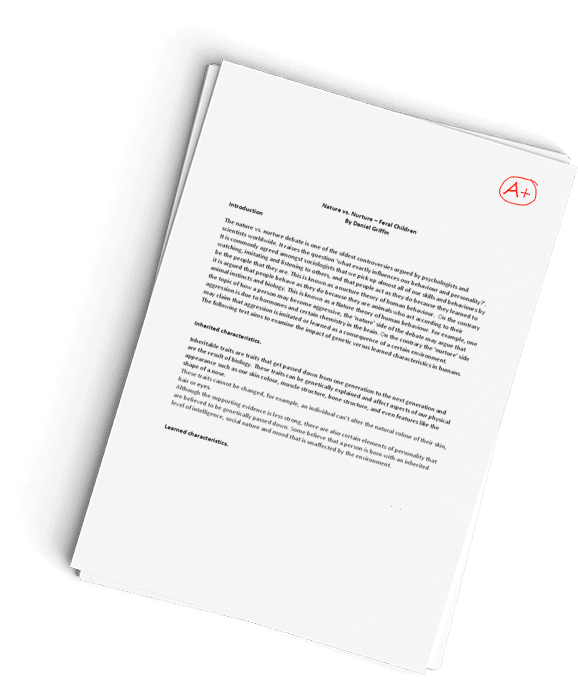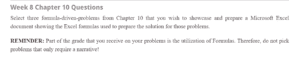Get a timely done, PLAGIARISM-FREE paper
from our highly-qualified writers!## Chapter 10 Questions

Sample Answer## Chapter 10 Questions

### Question 1

 Question 1 Price before dividend Price after dividend Dividend Tax on capital gains Tax on ordinary income 50 46.5 5 0.7 58.00%

To calculate the marginal tax rate on stockholders, we use the formula below:
(𝑃_𝐵−𝑃_𝐴)/𝐷𝑖𝑣𝑖𝑑𝑒𝑛𝑑=(1−𝑡_𝑜)/(1−𝑡_𝑐𝑔 )
Where:
𝑃_𝐵=𝑃𝑟𝑖𝑐𝑒 𝑏𝑒𝑓𝑜𝑟𝑒 𝑑𝑖𝑣𝑖𝑑𝑒𝑛𝑑
𝑃_𝐴=𝑃𝑟𝑖𝑐𝑒 𝑎𝑓𝑡𝑒𝑟 𝑑𝑖𝑣𝑖𝑑𝑒𝑛𝑑
𝑡_𝑜=𝑇𝑎𝑥 𝑜𝑛 𝑜𝑟𝑑𝑖𝑛𝑎𝑟𝑦 𝑖𝑛𝑐𝑜𝑚𝑒
𝑡_𝑐𝑔=𝑇𝑎𝑥 𝑜𝑛 𝑐𝑎𝑝𝑖𝑡𝑎𝑙 𝑔𝑎𝑖𝑛𝑠

Substituting the known values:
(50−46.5)/5=(1−𝑡_𝑜)/(1−0.4)
Re-arranging to make 𝑡_𝑜 the subject of the formula:
𝑡_𝑜=1−((50−46.5)(1−0.4)/5)
𝑡_𝑜=1−0.42
𝑡_𝑜=0.58=58%

### Question 3

 Question 3 Capital Gains Rate 50% Price Before Dividend Price After Dividend Dividend Tax on Capital Gains (1+R) (1+R)^n Number of years Ordinary Tax Rate 50% 10 9.2 1 37.50% 1.10 1.33 3 Discount Rate 10%

First, calculate the effective capital gain rate:

Effective capital gains rate=(Stated capital gains rate)/(1+R)^n

Where:

R=Discount rate=10%

n=Number of periods

Substituting the known values into the formula:

Effective capital gains rate=0.5/(1+0.1)^n =0.5/〖1.1〗^n

Next, relate the prices before and after dividend to taxes using the following formula:

(P_B-P_A)/Dividend=(1-t_o)/(1-t_cg )

Where:

P_B=Price before dividend=10

P_A=Price after dividend=9.2

t_o=Tax on ordinary income=50%

t_cg=Tax on capital gains=0.5/〖1.1〗^n

Substituting the values into the formula:

(10-9.2)/1.0=(1-0.5)/(1-0.5/〖1.1〗^n )

0.8=0.5/(1-0.5/〖1.1〗^n )

Simplifying the expressions to solve for n gives:

〖1.1〗^n=1.33

Solving for n using logarithms gives:

n≅3

The capital gains taxes are being deferred by 3 years.

### Question 4

 Question 4 Ordinary income tax rate 40% Effective Tax Rate on Dividends Price Before Dividend Dividend Price After Dividend Change in Share Price Capital gains tax rate 28% 6.00% 10 0.5 9.35 0.65 % of exempt dividend 85%

First, we evaluate the effective tax rate on dividends.
𝐸𝑓𝑓𝑒𝑐𝑡𝑖𝑣𝑒 𝑡𝑎𝑥 𝑟𝑎𝑡𝑒 𝑜𝑛 𝑑𝑖𝑣𝑖𝑑𝑒𝑛𝑑𝑠=(𝑇𝑎𝑥 𝑟𝑎𝑡𝑒 𝑜𝑛 𝑜𝑟𝑑𝑖𝑛𝑎𝑟𝑦 𝑖𝑛𝑐𝑜𝑚𝑒)(1−% 𝑜𝑓 𝑒𝑥𝑒𝑚𝑝 𝑑𝑖𝑣𝑖𝑑𝑒𝑛𝑑𝑠)

Where:

𝑂𝑟𝑑𝑖𝑛𝑎𝑟𝑦 𝑖𝑛𝑐𝑜𝑚𝑒 𝑡𝑎𝑥 𝑟𝑎𝑡𝑒=40%
𝐶𝑎𝑝𝑖𝑡𝑎𝑙 𝑔𝑎𝑖𝑛𝑠 𝑡𝑎𝑥 𝑟𝑎𝑡𝑒=28%
𝐷𝑖𝑣𝑖𝑑𝑒𝑛𝑑 𝑝𝑒𝑟 𝑠ℎ𝑎𝑟𝑒=\$0.50
% 𝑜𝑓 𝑒𝑥𝑒𝑚𝑝𝑡 𝑑𝑖𝑣𝑖𝑑𝑒𝑛𝑑𝑠=85%

Substituting the values into the equation.

𝐸𝑓𝑓𝑒𝑐𝑡𝑖𝑣𝑒 𝑡𝑎𝑥 𝑟𝑎𝑡𝑒 𝑜𝑛 𝑑𝑖𝑣𝑖𝑑𝑒𝑛𝑑𝑠=(0.4)(1−0.85)
𝐸𝑓𝑓𝑒𝑐𝑡𝑖𝑣𝑒 𝑡𝑎𝑥 𝑟𝑎𝑡𝑒 𝑜𝑛 𝑑𝑖𝑣𝑖𝑑𝑒𝑛𝑑𝑠=0.06=6%

Next, use the equation relating the prices before and after dividends to taxes to find the change in share price.

(𝑃_𝐵−𝑃_𝐴)/𝐷𝑖𝑣𝑖𝑑𝑒𝑛𝑑=(1−𝑡_𝑜)/(1−𝑡_𝑐𝑔 )

Where:

𝑃_𝐵=𝑃𝑟𝑖𝑐𝑒 𝑏𝑒𝑓𝑜𝑟𝑒 𝑑𝑖𝑣𝑖𝑑𝑒𝑛𝑑=\$10
𝑃_𝐴=𝑃𝑟𝑖𝑐𝑒 𝑎𝑓𝑡𝑒𝑟 𝑑𝑖𝑣𝑖𝑑𝑒𝑛𝑑
𝑡_𝑜=𝑇𝑎𝑥 𝑜𝑛 𝑜𝑟𝑑𝑖𝑛𝑎𝑟𝑦 𝑖𝑛𝑐𝑜𝑚𝑒=6%(𝐴𝑓𝑡𝑒𝑟 𝑎𝑑𝑗𝑢𝑠𝑡𝑚𝑒𝑛𝑡)
𝑡_𝑐𝑔=𝑇𝑎𝑥 𝑜𝑛 𝑐𝑎𝑝𝑖𝑡𝑎𝑙 𝑔𝑎𝑖𝑛𝑠=28%

Substituting the given and calculated values into the equation.

(\$10−𝑃_𝐴)/\$0.50=(1−0.06)/(1−0.28)
Making 𝑃_𝐴 the subject of the equation.
𝑃_𝐴=\$10−((1−0.06)(\$0.50)/((1−0.28) ))
𝑃_𝐴=\$10−\$0.653
𝑃_𝐴≅9.35

The share price drops from \$10 to \$9.35 after the dividend.

## Reference

Damodaran, A. (2010). Applied corporate finance. John Wiley & Sons.

ORDER A PLAGIARISM-FREE PAPER HERE

We’ll write everything from scratch

Question## Week 8 Chapter 10 Questions

Select three formula-driven-problems from Chapter 10 that you wish to showcase and prepare a Microsoft Excel document showing the Excel formulas used to prepare the solution for those problems.Chapter 10 Questions

REMINDER: Part of the grade that you receive on your problems is the utilization of Formulas. Therefore, do not pick problems that only require a narrative!

Have a similar assignment? "Place an order for your assignment and have exceptional work written by our team of experts, guaranteeing you A results."## Our Service Charter

1. Professional & Expert Writers: Eminence Papers only hires the best. Our writers are specially selected and recruited, after which they undergo further training to perfect their skills for specialization purposes. Moreover, our writers are holders of masters and Ph.D. degrees. They have impressive academic records, besides being native English speakers.

2. Top Quality Papers: Our customers are always guaranteed of papers that exceed their expectations. All our writers have +5 years of experience. This implies that all papers are written by individuals who are experts in their fields. In addition, the quality team reviews all the papers before sending them to the customers.

3. Plagiarism-Free Papers: All papers provided by Eminence Papers are written from scratch. Appropriate referencing and citation of key information are followed. Plagiarism checkers are used by the Quality assurance team and our editors just to double-check that there are no instances of plagiarism.

4. Timely Delivery: Time wasted is equivalent to a failed dedication and commitment. Eminence Papers are known for the timely delivery of any pending customer orders. Customers are well informed of the progress of their papers to ensure they keep track of what the writer is providing before the final draft is sent for grading.

5. Affordable Prices: Our prices are fairly structured to fit in all groups. Any customer willing to place their assignments with us can do so at very affordable prices. In addition, our customers enjoy regular discounts and bonuses.

6. 24/7 Customer Support: At Eminence Papers, we have put in place a team of experts who answer all customer inquiries promptly. The best part is the ever-availability of the team. Customers can make inquiries anytime.